International
Tables for
Crystallography
Volume B
Reciprocal space
Edited by U. Shmueli

International Tables for Crystallography (2010). Vol. B, ch. 1.3, p. 28   | 1 | 2 |

## Section 1.3.2.2.6.2. Topological vector spaces

G. Bricognea

aGlobal Phasing Ltd, Sheraton House, Suites 14–16, Castle Park, Cambridge CB3 0AX, England, and LURE, Bâtiment 209D, Université Paris-Sud, 91405 Orsay, France

#### 1.3.2.2.6.2. Topological vector spaces

| top | pdf |

The function spaces E of interest in Fourier analysis have an underlying vector space structure over the fieldof complex numbers. A topology on E is said to be compatible with a vector space structure on E if vector addition [i.e. the map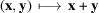] and scalar multiplication [i.e. the map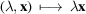] are both continuous; E is then called a topological vector space. Such a topology may be defined by specifying a `fundamental system S of neighbourhoods of', which can then be translated by vector addition to construct neighbourhoods of other points.

A norm ν on a vector space E is a non-negative real-valued function onsuch that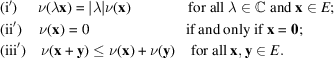Subsets of E defined by conditions of the formwith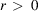form a fundamental system of neighbourhoods of 0. The corresponding topology makes E a normed space. This topology is metrizable, since it is equivalent to that derived from the translation-invariant distance. Normed spaces which are complete, i.e. in which all Cauchy sequences converge, are called Banach spaces; they constitute the natural setting for the study of differential calculus.

A semi-norm σ on a vector space E is a positive real-valued function onwhich satisfies (i′) and (iii′) but not (ii′). Given a set Σ of semi-norms on E such that any pair (x, y) inis separated by at least one, let B be the set of those subsetsof E defined by a condition of the formwithand; and let S be the set of finite intersections of elements of B. Then there exists a unique topology on E for which S is a fundamental system of neighbourhoods of 0. This topology is uniformizable since it is equivalent to that derived from the family of translation-invariant pseudo-distances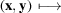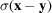. It is metrizable if and only if it can be constructed by the above procedure with Σ a countable set of semi-norms. If furthermore E is complete, E is called a Fréchet space.

If E is a topological vector space over, its dualis the set of all linear mappings from E to(which are also called linear forms, or linear functionals, over E). The subspace ofconsisting of all linear forms which are continuous for the topology of E is called the topological dual of E and is denoted E′. If the topology on E is metrizable, then the continuity of a linear formatcan be ascertained by means of sequences, i.e. by checking that the sequence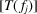of complex numbers converges to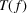inwhenever the sequence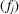converges to f in E.